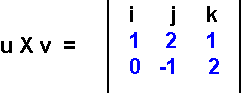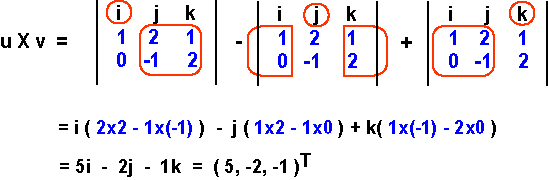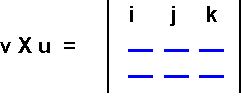What is the cross product of (1, 2, 1)T with (0, -1, 2)T ?# Evaluating the Determinant-like Thing

To evaluate this, find the cofactors of i, j, k in the top row. In the picture, the cofactors are circled in red. The cofactor for i, for instance, is the elements of rows 2 and 3 other than those in column i.To evaluate a cofactor, multiply the two elements on the main diagonal, subtract from that the product of the two elements on the other diagonal. For example, for the cofactor of i this is 2×2 - 1×(-1)  =  2 + 1  = 5

### QUESTION 13:

What is the cross product of (0, -1, 2)T with (1, 2, 1)T ?   Fill in the blanks. (NOTE: the order of the vectors has been reversed).